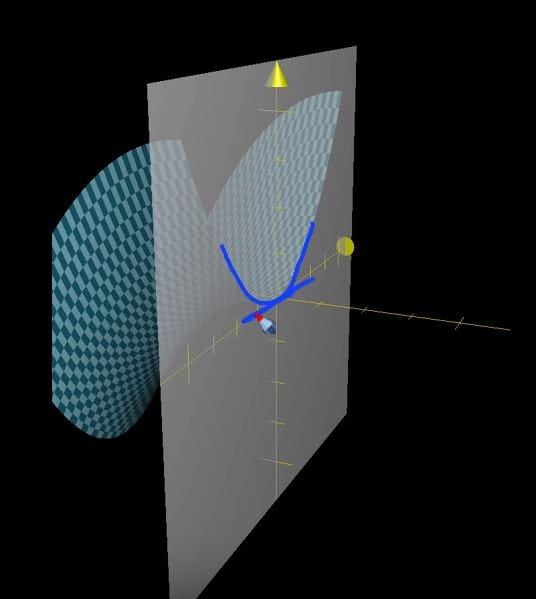# The Dilemma of High Dimensional Calculus

In machine Learning , we propose multi-variable function as hypothesis. The problem arises when we have to find global minimum of that function because these multi-variant functions have a lot of valleys and hills,and it becomes really difficult to find the global minimum if you get stuck in one of these regions.But,the most dangerous regions in a multi-variable function are saddle points where you don’t know whether this point is a minima or maxima but still that point is a critical point(will come to this in some time).

In this post , we will discuss the whole process of finding points of minima or maxima of a multi-variable function by intuitively understanding the saddle points and why they are dangerous.

For ease of writing and your understanding, let me set some notation reference

1. Partial derivative of f(x,y) w.r.t to x = Fx
2. Partial derivative of f(x,y) w.r.t to y = Fy
3. Second derivative of f(x,y ) w.r.t to x= Fxx
4. Second derivative of f(x,y ) w.rt. to y= Fyy
5. Second derivative of f(x,y) w.r.t to xy = Fxy

Let’s take the function, f(x,y) = x² - y²,

This is the plot of f(x,y) and we will evaluate the gradient at (0,0).

The partial derivative of f(x,y) w.r.t to x = 2x and w.r.t to y is -2y.

The value at (0,0) is 0 for partial derivative x and 0 for partial derivative y.

Now let’s view the graph from each of the partial derivative perspective.Partial derviative w.r.t y ( x constant)

Above we see ,the graph is a point of local maxima at (0,0).partial derivative w.r.t to x (y constant)

Here we see , it seems like it is a point of local minima.

It is pretty much evident that Fx(x,y) = 2x (up facing parabola) and Fy(x,y) = -2y(downwards parabola) disagree with each other and the point (0,0) is a saddle point.This concept of saddle point is also named after its shape of a saddle.But, how to we find about these dilemma-tic regions.

Let’s go throught the whole process of finding out whether a given point is a point of minima or maxima :

1. First, we find all the critical points.The critical points are points where slope is zero or in general the candidate points which could be minimum or maximum.
2. Secondly, We find the Fxx and Fyy and Fxy at critical points.
3. Let’s define determining term , H = (Fxx*Fyy)-(Fxy)²

if H < 0 , it is a saddle point

if H>0, it is either a minima or maxima

if H=0, then we are really in a bad situation and cannot determine it.

When H>0, in that case (Fxy)² < (Fxx)(Fyy) and in that case, there are two cases when both Fxx and Fyy are positive, in that case, it is a point of minima.Similarly,if Fxx and Fyy are negative then they both are in strong correlation to call it a point of maxima.

When H<0, clearly there are two cases again , either the Fxx and Fyy are in disagreement ,means both have different sign and fxy is positive or negative.Other case is, when Fxx and Fyy are having same sign but still (Fxy)² has greater magnitude than Fxx*Fyy.

What we observe is that,Fxy playes a crucial role in deciding whether a point is a saddle point or a point of maxima or minima.

But here is the problem, computing second order derivatives of the weights in the objective function(hypothesis) is computationally not feasible.Therefore, we cannot use the second order test(determining H) in real time implementation so that we cannot get trapped in a saddle point. Rather stochastic gradient descent is used , which is a first order derivative technique for the optimal minimum.

Above , i had taken example of a function which is has a 2D input. In case of N dimensional input, we use eigendecomposition of Hessian matrix.

Following are the condition on hessian matrix :

1. If Hessian is a positive definite matrix (all eigen values positive), then it is a point of local minima.
2. If Hessian is a negative definite matrix (all eigen values positive), then it is a point of local maxima.
3. If one eigen value is positive and atleast one is negative,then x is a local maxima on one of the cross-section of f but a local minima on another cross-section.
4. This test fails , when all non-zero eigen values have same sign but at least one eigen value is zero.

The saddle points are very unstable and are very difficult to escape with computational limitation we have right now.Newton’s method is second order descent algorithm like gradient descent which can help overcome this problem but as i mentioned above, it is not computationally feasible.

--

--